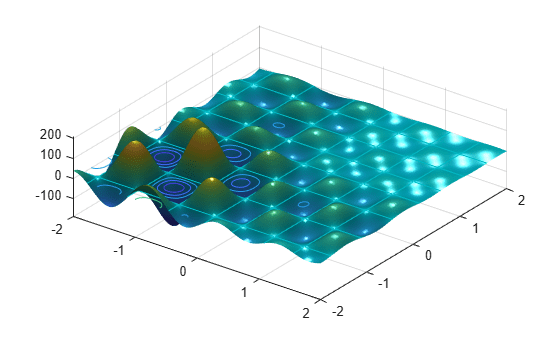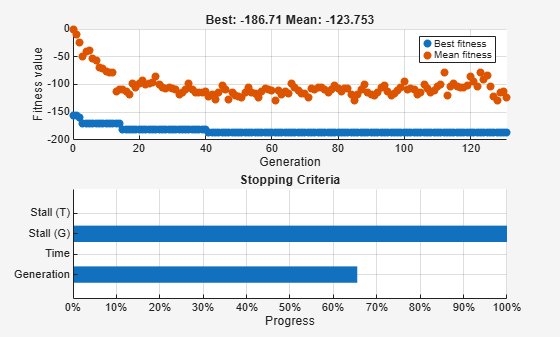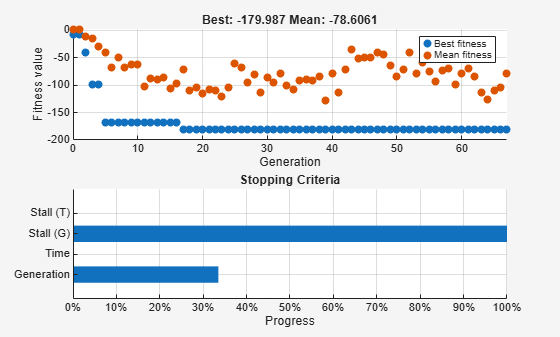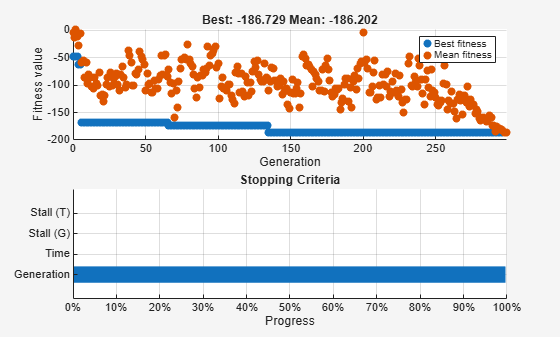Main Content

# Effects of Genetic Algorithm Options

This example shows the effects of some options for the genetic algorithm function `ga`. You create and change options by using the `optimoptions` function.

### Set Up a Problem for `ga`

`ga` searches for a minimum of a function using the genetic algorithm. For this example, use `ga` to minimize the fitness function `shufcn`, a real-valued function of two variables.

Plot `shufcn` over the range `= [-2 2;-2 2]` by calling `plotobjective`.

`plotobjective(@shufcn,[-2 2; -2 2]);`To use the `ga` solver, provide at least two input arguments: a fitness function and the number of variables in the problem. The first two output arguments returned by `ga` are `x`, the best point found, and `Fval`, the function value at the best point. A third output argument, `exitFlag`, indicates why `ga` stopped. `ga` can also return a fourth argument, `Output`, which contains information about the performance of the solver.

```FitnessFunction = @shufcn; numberOfVariables = 2;```

Run the `ga` solver.

```rng default % For reproducibility [x,Fval,exitFlag,Output] = ga(FitnessFunction,numberOfVariables);```
```Optimization terminated: average change in the fitness value less than options.FunctionTolerance. ```
`fprintf('The number of generations is: %d\n', Output.generations);`
```The number of generations is: 124 ```
`fprintf('The number of function evaluations is: %d\n', Output.funccount);`
```The number of function evaluations is: 5881 ```
`fprintf('The best function value found is: %g\n', Fval);`
```The best function value found is: -186.199 ```

If you run this example without the `rng default` command, your results can differ, because `ga` is a stochastic algorithm.

### How the Genetic Algorithm Works

The genetic algorithm works on a population using a set of operators that are applied to the population. A population is a set of points in the design space. The initial population is generated randomly by default. The algorithm computes the next generation of the population using the fitness of the individuals in the current generation. For details, see How the Genetic Algorithm Works.

### Add Visualization

To visualize the solver performance while it is running, set a `'PlotFcn'` option using `optimoptions`. In this case, select two plot functions in a cell array. Set `gaplotbestf`, which plots the best and mean score of the population at every generation. Also set `gaplotstopping`, which plots the percentage of stopping criteria satisfied.

`opts = optimoptions(@ga,'PlotFcn',{@gaplotbestf,@gaplotstopping});`

Run the `ga` solver, including the `opts` argument.

```[x,Fval,exitFlag,Output] = ... ga(FitnessFunction,numberOfVariables,[],[],[],[],[],[],[],opts);```
```Optimization terminated: average change in the fitness value less than options.FunctionTolerance. ```### Specify Population Options

Population options can have a large effect on solver performance. The speed of each iteration depends on the population size: a larger population leads to slower iterations. Conversely, a larger population leads to `ga` exploring more thoroughly, so can lead to a better solution. Similarly, a wider initial range can lead to more thorough exploration, but can require a larger population to explore the wider range with a similar thoroughness.

Specify Population Size

`ga` creates a default initial population by using a uniform random number generator. The default population size used by `ga` is 50 when the number of decision variables is less than 5, and 200 otherwise. The default size might not work well for some problems; for example, a smaller population size can be sufficient for smaller problems. Since the current problem has only two variables, specify a population size of 10. Set the value of the option `PopulationSize` to 10 in the existing options, `opts`.

`opts.PopulationSize = 10;`

Specify Initial Population Range

The default method for generating an initial population uses a uniform random number generator. For problems without integer constraints, `ga` creates an initial population where all the points are in the range –10 to 10. For example, you can generate a population of size three in the default range using this command:

`Population = [-10,-10] + 20*rand(3,2);`

You can set the initial range by changing the `InitialPopulationRange` option. The range must be a matrix with two rows. If the range has only one column, that is, it is 2-by-1, then the range of every variable is the given range. For example, if you set the range to `[-1; 1]`, then the initial range for both variables is –1 to 1. To specify a different initial range for each variable, you must specify the range as a matrix with two rows and `numberOfVariables` columns. For example, if you set the range to `[-1 0; 1 2]`, then the first variable has the range –1 to 1, and the second variable has the range 0 to 2 (each column corresponds to a variable).

Modify the value of the option `InitialPopulationRange` in the existing options, `opts`.

`opts.InitialPopulationRange = [-1 0; 1 2];`

Run the `ga` solver.

```[x,Fval,exitFlag,Output] = ga(FitnessFunction,numberOfVariables,[],[],[], ... [],[],[],[],opts);```
```Optimization terminated: average change in the fitness value less than options.FunctionTolerance. ````fprintf('The number of generations is: %d\n', Output.generations);`
```The number of generations is: 67 ```
`fprintf('The number of function evaluations is: %d\n', Output.funccount);`
```The number of function evaluations is: 614 ```
`fprintf('The best function value found is: %g\n', Fval);`
```The best function value found is: -179.987 ```

### Reproduce Results

By default, `ga` starts with a random initial population created using MATLAB® random number generators. The solver produces the next generation using `ga` operators that also use these same random number generators. Every time a random number is generated, the state of the random number generators changes. So, even if you do not change any options, you can get different results when you run the solver again.

Run the solver twice to show this phenomenon.

Run the `ga` solver.

`[x,Fval,exitFlag,Output] = ga(FitnessFunction,numberOfVariables);`
```Optimization terminated: average change in the fitness value less than options.FunctionTolerance. ```
`fprintf('The best function value found is: %g\n', Fval);`
```The best function value found is: -186.484 ```

Run `ga` again.

`[x,Fval,exitFlag,Output] = ga(FitnessFunction,numberOfVariables);`
```Optimization terminated: average change in the fitness value less than options.FunctionTolerance. ```
`fprintf('The best function value found is: %g\n', Fval);`
```The best function value found is: -185.867 ```

`ga` gives different results in the two runs because the state of the random number generator changes from one run to another.

If you want to reproduce your results before you run `ga`, you can save the state of the random number stream.

`thestate = rng;`

Run `ga`.

`[x,Fval,exitFlag,Output] = ga(FitnessFunction,numberOfVariables);`
```Optimization terminated: average change in the fitness value less than options.FunctionTolerance. ```
`fprintf('The best function value found is: %g\n', Fval);`
```The best function value found is: -186.467 ```

Reset the stream and rerun `ga`. The results are identical to the previous run.

```rng(thestate); [x,Fval,exitFlag,Output] = ga(FitnessFunction,numberOfVariables);```
```Optimization terminated: average change in the fitness value less than options.FunctionTolerance. ```
`fprintf('The best function value found is: %g\n', Fval);`
```The best function value found is: -186.467 ```

If you run `ga` before specifying to reproduce the results, you can reset the random number generator as long as you have the `output` structure.

```strm = RandStream.getGlobalStream; strm.State = Output.rngstate.State;```

Rerun `ga`. Again, the results are identical.

`[x,Fval,exitFlag,Output] = ga(FitnessFunction,numberOfVariables);`
```Optimization terminated: average change in the fitness value less than options.FunctionTolerance. ```
`fprintf('The best function value found is: %g\n', Fval);`
```The best function value found is: -186.467 ```

### Modify Stopping Criteria

`ga` uses four different criteria to determine when to stop the solver. `ga` stops when it reaches the maximum number of generations; by default, this number is 100 times the number of variables. `ga` also detects if the best fitness value does not change for some time given in seconds (stall time limit), or for some number of generations (maximum stall generations). Another criteria is the maximum time limit in seconds. Modify the stopping criteria to increase the maximum number of generations to 300 and the maximum stall generations to 100.

`opts = optimoptions(opts,'MaxGenerations',300,'MaxStallGenerations', 100);`

Rerun the `ga` solver.

```[x,Fval,exitFlag,Output] = ga(FitnessFunction,numberOfVariables,[],[],[], ... [],[],[],[],opts);```
```Optimization terminated: average change in the fitness value less than options.FunctionTolerance. ````fprintf('The number of generations is: %d\n', Output.generations);`
```The number of generations is: 299 ```
`fprintf('The number of function evaluations is: %d\n', Output.funccount);`
```The number of function evaluations is: 2702 ```
`fprintf('The best function value found is: %g\n', Fval);`
```The best function value found is: -186.729 ```

### Specify `ga` Operators

`ga` starts with a random set of points in the population and uses operators to produce the next generation of the population. The different operators are scaling, selection, crossover, and mutation. The toolbox provides several functions to specify for each operator. Specify `fitscalingprop` for `FitnessScalingFcn` and `selectiontournament` for `SelectionFcn`.

```opts = optimoptions(@ga,'SelectionFcn',@selectiontournament, ... 'FitnessScalingFcn',@fitscalingprop);```

Rerun `ga`.

```[x,Fval,exitFlag,Output] = ga(FitnessFunction,numberOfVariables,[],[],[], ... [],[],[],[],opts);```
```Optimization terminated: average change in the fitness value less than options.FunctionTolerance. ```
`fprintf('The number of generations is: %d\n', Output.generations);`
```The number of generations is: 52 ```
`fprintf('The number of function evaluations is: %d\n', Output.funccount);`
```The number of function evaluations is: 2497 ```
`fprintf('The best function value found is: %g\n', Fval);`
```The best function value found is: -186.417 ```

The best function value can improve or get worse based on the specified operators. Experimenting with different operators is often the best way to determine which set of operators works best for your problem.

Watch now